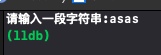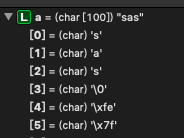2019-09-10 16:25

# 求助！为什么在c语言中利用scanf将字符串写入数组中会出现缺少第一个字符的情况

``````#include <stdio.h>

int main() {
char a;        //设置一个容量为100的数组存放用户所要输入的值
int cnt = 0;        //定义一个计数器
int j = 0;
char ch;
printf("请输入一段字符串:");        //提示语
while ((ch = getchar()) != '\n') {      //设置一个while循环，当用户输入回车的时候就跳出循环
scanf("%s", a);                 //将用户输入的字符串放在数组a当中
for (j = 0; j < 100; j++) {     //使用for循环来一一检查是否有as
if (a[j] == 'a' && a[j+1] == 's') {
cnt++;                  //如果满足条件，计数器加一
}
}
}
printf("%d\n", cnt);          //最后打印出结果
}
``````• 写回答
• 关注问题
• 收藏
• 邀请回答

#### 3条回答默认 最新

•qtchen_1988 2019-09-10 17:02
已采纳

getchar 会吃掉字符

``````#include <stdio.h>

int main() {
char a = {};        //设置一个容量为100的数组存放用户所要输入的值
int cnt = 0;        //定义一个计数器
int j = 0;
char ch;
printf("请输入一段字符串:");   //提示语
int i = 0;
while ((ch = getchar()) != '\n') {      //设置一个while循环，当用户输入回车的时候就跳出循环
a[i++] = ch;               //将用户输入的字符串放在数组a当中
}
for (j = 0; j < 100; j++) {     //使用for循环来一一检查是否有as
if (a[j] == 'a' && a[j+1] == 's') {
cnt++;                  //如果满足条件，计数器加一
}
}
printf("%d\n", cnt);          //最后打印出结果
}
``````
打赏 评论
•getchar()会读取你输入的第一个字符
第一次getchar()的返回值就是'a'
所以数组a中没有'a'

打赏 评论
•大头萝卜 2019-09-10 17:08

代码有问题。应该先读字符串，再判断。代码如下修改即可。

#include

int main() {
char a; //设置一个容量为100的数组存放用户所要输入的值
int cnt = 0; //定义一个计数器
int j = 0;
char ch;
printf("请输入一段字符串:"); //提示语
do
{
scanf("%s", a); //将用户输入的字符串放在数组a当中
for (j = 0; j < 100; j++) { //使用for循环来一一检查是否有as
if (a[j] == 'a' && a[j+1] == 's') {
cnt++; //如果满足条件，计数器加一
}
}
}
while ((ch = getchar()) != '\n'); //当用户直接输入回车的时候就跳出循环
printf("%d\n", cnt); //最后打印出结果
}

打赏 评论